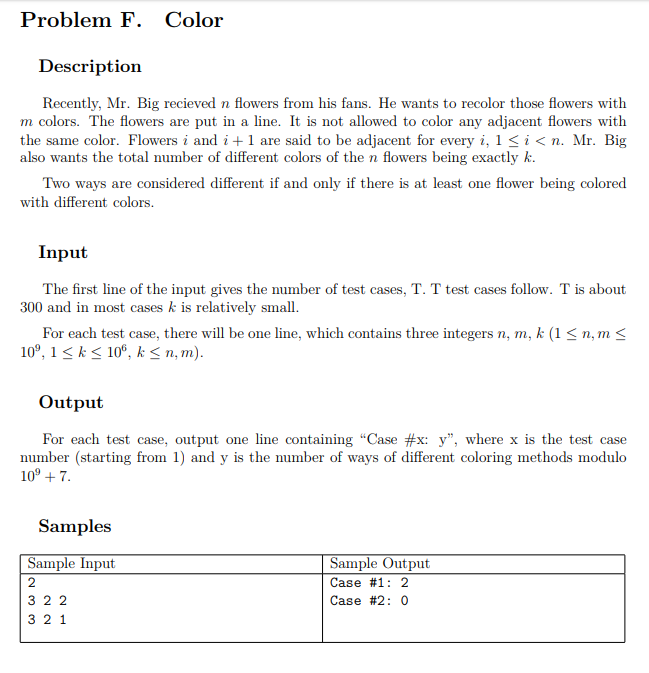# $\large\mathcal{Description}$$\Large{☞}$ 提交通道 ($F$ 题)

# $\large\mathcal{Solution}$

$k \times (k - 1)^{n-1}$ 是至多选 $k$ 种的方案数，而不是恰好选 $k$ 种的方案数。